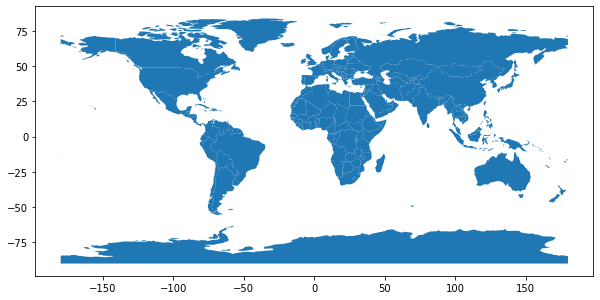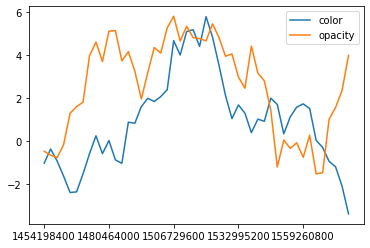# Introduction¶

In this notebook we'll make a choropleth with a timeslider. We'll use my branched version of folium, which contains a plugin with a class called TimeDynamicGeoJson.

The class needs at least two arguments to be instantiated.

1. A string-serielized geojson containing all the features (i.e., the areas)
2. A dictionary with the following structure:
styledict = {
'0': {
'2017-1-1': {'color': 'ffffff', 'opacity': 1}
'2017-1-2': {'color': 'fffff0', 'opacity': 1}
...
},
...,
'n': {
'2017-1-1': {'color': 'ffffff', 'opacity': 1}
'2017-1-2': {'color': 'fffff0', 'opacity': 1}
...
}
}


In the above dictionary, the keys are the feature-ids.

Using both color and opacity gives us the ability to simultaneously visualize two features on the choropleth. I typically use color to visualize the main feature (like, average height) and opacity to visualize how many measurements were in that group.

We use geopandas to load a dataset containing the boundaries of all the countries in the world.

In :
import geopandas as gpd

assert "naturalearth_lowres" in gpd.datasets.available
datapath = gpd.datasets.get_path("naturalearth_lowres")

In :
%matplotlib inline

ax = gdf.plot(figsize=(10, 10))The GeoDataFrame contains the boundary coordinates, as well as some other data such as estimated population.

In :
gdf.head()

Out:
pop_est continent name iso_a3 gdp_md_est geometry
0 920938 Oceania Fiji FJI 8374.0 MULTIPOLYGON (((180.00000 -16.06713, 180.00000...
1 53950935 Africa Tanzania TZA 150600.0 POLYGON ((33.90371 -0.95000, 34.07262 -1.05982...
2 603253 Africa W. Sahara ESH 906.5 POLYGON ((-8.66559 27.65643, -8.66512 27.58948...
3 35623680 North America Canada CAN 1674000.0 MULTIPOLYGON (((-122.84000 49.00000, -122.9742...
4 326625791 North America United States of America USA 18560000.0 MULTIPOLYGON (((-122.84000 49.00000, -120.0000...

## Creating the style dictionary¶

Now we generate time series data for each country.

Data for different areas might be sampled at different times, and TimeDynamicGeoJson can deal with that. This means that there is no need to resample the data, as long as the number of datapoints isn't too large for the browser to deal with.

To simulate that data is sampled at different times we random sample data for n_periods rows of data and then pick without replacing n_sample of those rows.

In :
import pandas as pd

n_periods, n_sample = 48, 40

assert n_sample < n_periods

datetime_index = pd.date_range("2016-1-1", periods=n_periods, freq="M")
dt_index_epochs = datetime_index.astype(int) // 10 ** 9
dt_index = dt_index_epochs.astype("U10")

dt_index

Out:
Index(['1454198400', '1456704000', '1459382400', '1461974400', '1464652800',
'1467244800', '1469923200', '1472601600', '1475193600', '1477872000',
'1480464000', '1483142400', '1485820800', '1488240000', '1490918400',
'1493510400', '1496188800', '1498780800', '1501459200', '1504137600',
'1506729600', '1509408000', '1512000000', '1514678400', '1517356800',
'1519776000', '1522454400', '1525046400', '1527724800', '1530316800',
'1532995200', '1535673600', '1538265600', '1540944000', '1543536000',
'1546214400', '1548892800', '1551312000', '1553990400', '1556582400',
'1559260800', '1561852800', '1564531200', '1567209600', '1569801600',
'1572480000', '1575072000', '1577750400'],
dtype='object')
In :
import numpy as np

styledata = {}

for country in gdf.index:
df = pd.DataFrame(
{
"color": np.random.normal(size=n_periods),
"opacity": np.random.normal(size=n_periods),
},
index=dt_index,
)
df = df.cumsum()
df.sample(n_sample, replace=False).sort_index()
styledata[country] = df


Note that the geodata and random sampled data is linked through the feature_id, which is the index of the GeoDataFrame.

In :
gdf.loc

Out:
pop_est                                                  920938
continent                                               Oceania
name                                                       Fiji
iso_a3                                                      FJI
gdp_md_est                                                 8374
geometry      (POLYGON ((180 -16.06713266364245, 180 -16.555...
Name: 0, dtype: object
In :
styledata.get(0).head()

Out:
color opacity
1454198400 1.224453 -1.836594
1456704000 1.854374 -1.282901
1459382400 1.436914 -2.552985
1461974400 1.361031 -2.401590
1464652800 1.879093 -0.972160

We see that we generated two series of data for each country; one for color and one for opacity. Let's plot them to see what they look like.

In :
ax = df.plot()Looks random alright. We want to map the column named color to a hex color. To do this we use a normal colormap. To create the colormap, we calculate the maximum and minimum values over all the timeseries. We also need the max/min of the opacity column, so that we can map that column into a range [0,1].

In :
max_color, min_color, max_opacity, min_opacity = 0, 0, 0, 0

for country, data in styledata.items():
max_color = max(max_color, data["color"].max())
min_color = min(max_color, data["color"].min())
max_opacity = max(max_color, data["opacity"].max())
max_opacity = min(max_color, data["opacity"].max())


Define and apply maps:

In :
from branca.colormap import linear

cmap = linear.PuRd_09.scale(min_color, max_color)

def norm(x):
return (x - x.min()) / (x.max() - x.min())

for country, data in styledata.items():
data["color"] = data["color"].apply(cmap)
data["opacity"] = norm(data["opacity"])

In :
styledata.get(0).head()

Out:
color opacity
1454198400 #ddcbe4ff 0.468719
1456704000 #d9c3dfff 0.540068
1459382400 #dcc9e2ff 0.376406
1461974400 #dccae3ff 0.395914
1464652800 #d9c3dfff 0.580110

Finally we use pd.DataFrame.to_dict() to convert each dataframe into a dictionary, and place each of these in a map from country id to data.

In :
styledict = {
str(country): data.to_dict(orient="index") for country, data in styledata.items()
}


Finally we can create the choropleth. I like to use the Stamen Toner tileset because its monochrome colors makes it neutral and clean looking.

If the time slider above doesn't show up in the notebook, it's probably because the output is being cropped. Try loading the saved .html file in your browser for the fullscreen experience.

In :
import folium
from folium.plugins import TimeSliderChoropleth

m = folium.Map([0, 0], tiles="Stamen Toner", zoom_start=2)

g = TimeSliderChoropleth(
gdf.to_json(),
styledict=styledict,